Pandas GroupBy

• Difficulty Level : Basic
• Last Updated : 29 Dec, 2021

Groupby is a pretty simple concept. We can create a grouping of categories and apply a function to the categories. It’s a simple concept but it’s an extremely valuable technique that’s widely used in data science. In real data science projects, you’ll be dealing with large amounts of data and trying things over and over, so for efficiency, we use Groupby concept. Groupby concept is really important because it’s ability to aggregate data efficiently, both in performance and the amount code is magnificent. Groupby mainly refers to a process involving one or more of the following steps they are:

• Splitting : It is a process in which we split data into group by applying some conditions on datasets.
• Applying : It is a process in which we apply a function to each group independently
• Combining : It is a process in which we combine different datasets after applying groupby and results into a data structure

The following image will help in understanding a process involve in Groupby concept.
1. Group the unique values from the Team column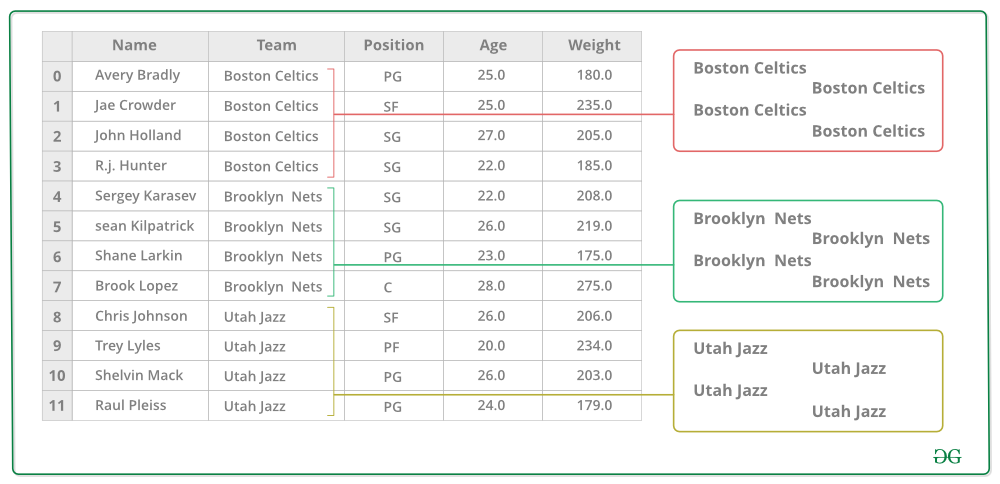2. Now there’s a bucket for each group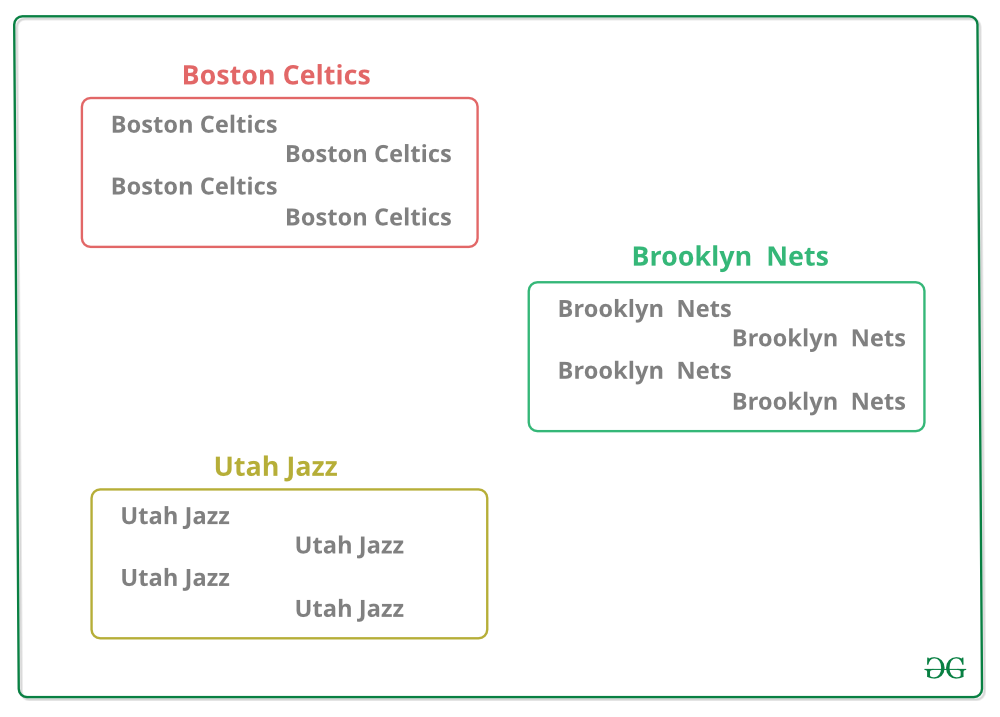3. Toss the other data into the buckets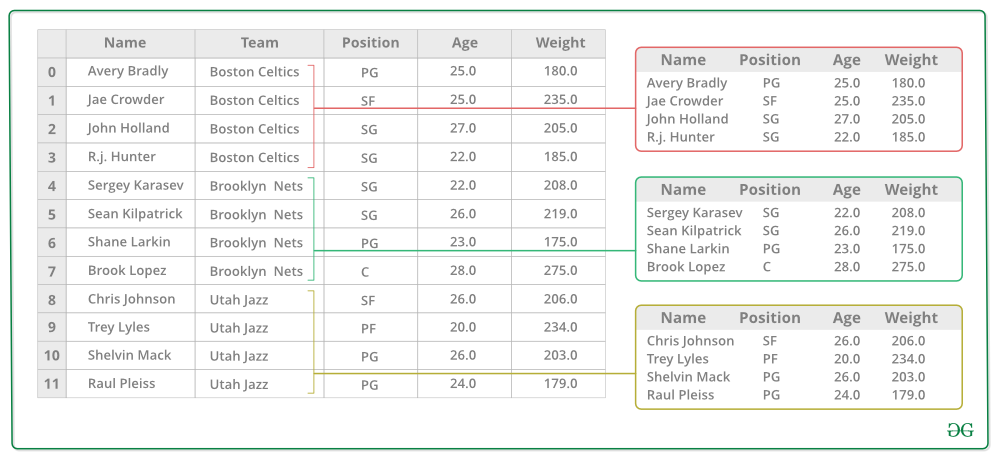4. Apply a function on the weight column of each bucket.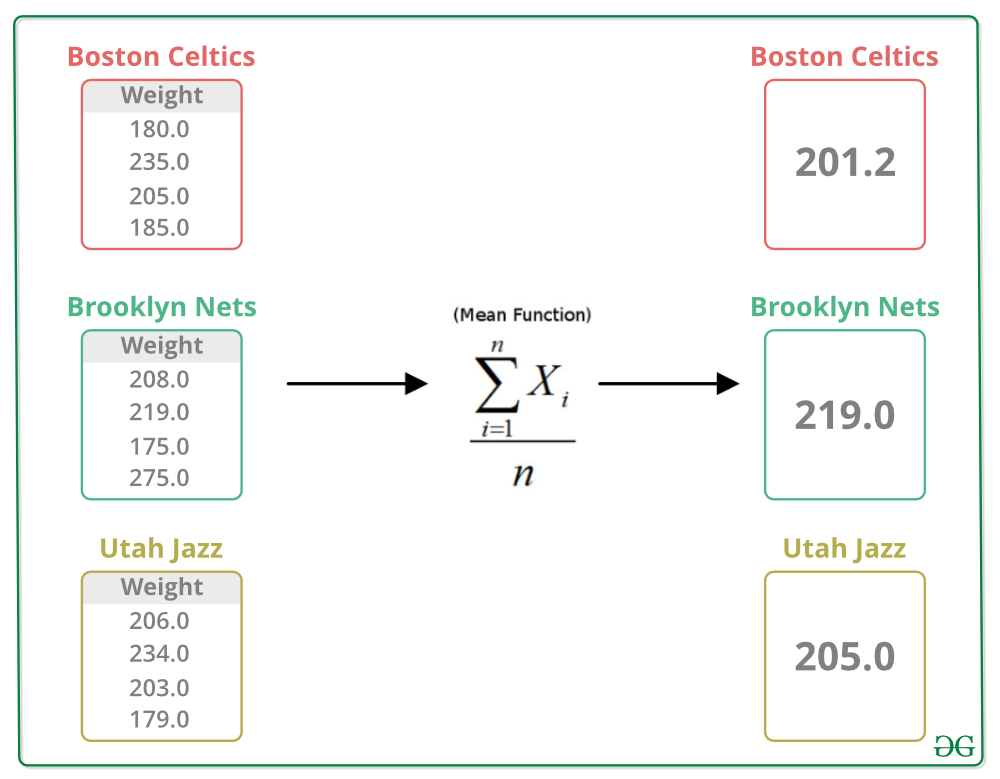Splitting Data into Groups

Splitting is a process in which we split data into a group by applying some conditions on datasets. In order to split the data, we apply certain conditions on datasets. In order to split the data, we use groupby() function this function is used to split the data into groups based on some criteria. Pandas objects can be split on any of their axes. The abstract definition of grouping is to provide a mapping of labels to group names. Pandas datasets can be split into any of their objects. There are multiple ways to split data like:

• obj.groupby(key)
• obj.groupby(key, axis=1)
• obj.groupby([key1, key2])

Note :In this we refer to the grouping objects as the keys.
Grouping data with one key:
In order to group data with one key, we pass only one key as an argument in groupby function.

Python3

 # importing pandas module import pandas as pd    # Define a dictionary containing employee data data1 = {'Name':['Jai', 'Anuj', 'Jai', 'Princi',                  'Gaurav', 'Anuj', 'Princi', 'Abhi'],         'Age':[27, 24, 22, 32,                33, 36, 27, 32],         'Address':['Nagpur', 'Kanpur', 'Allahabad', 'Kannuaj',                    'Jaunpur', 'Kanpur', 'Allahabad', 'Aligarh'],         'Qualification':['Msc', 'MA', 'MCA', 'Phd',                          'B.Tech', 'B.com', 'Msc', 'MA']}         # Convert the dictionary into DataFrame  df = pd.DataFrame(data1)    print(df)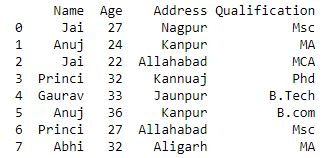Now we group a data of Name using groupby() function.

Python3

 # using groupby function # with one key   df.groupby('Name') print(df.groupby('Name').groups)

Output :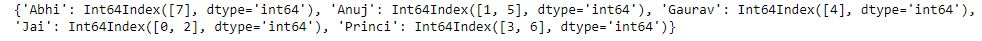Now we print the first entries in all the groups formed.

Python3

 # applying groupby() function to # group the data on Name value. gk = df.groupby('Name')     # Let's print the first entries # in all the groups formed. gk.first()

Output :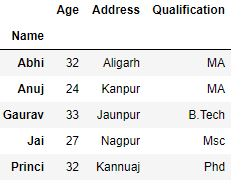Grouping data with multiple keys :
In order to group data with multiple keys, we pass multiple keys in groupby function.

Python3

 # importing pandas module import pandas as pd    # Define a dictionary containing employee data data1 = {'Name':['Jai', 'Anuj', 'Jai', 'Princi',                  'Gaurav', 'Anuj', 'Princi', 'Abhi'],         'Age':[27, 24, 22, 32,                33, 36, 27, 32],         'Address':['Nagpur', 'Kanpur', 'Allahabad', 'Kannuaj',                    'Jaunpur', 'Kanpur', 'Allahabad', 'Aligarh'],         'Qualification':['Msc', 'MA', 'MCA', 'Phd',                          'B.Tech', 'B.com', 'Msc', 'MA']}         # Convert the dictionary into DataFrame  df = pd.DataFrame(data1)    print(df)Now we group a data of “Name” and “Qualification” together using multiple keys in groupby function.

Python3

 # Using multiple keys in # groupby() function df.groupby(['Name', 'Qualification'])   print(df.groupby(['Name', 'Qualification']).groups)

Output :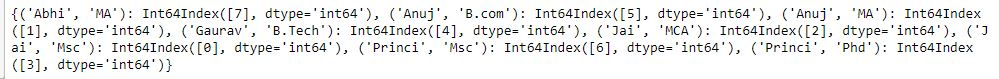Grouping data by sorting keys :
Group keys are sorted by default using the groupby operation. User can pass sort=False for potential speedups.

Python3

 # importing pandas module import pandas as pd    # Define a dictionary containing employee data data1 = {'Name':['Jai', 'Anuj', 'Jai', 'Princi',                  'Gaurav', 'Anuj', 'Princi', 'Abhi'],         'Age':[27, 24, 22, 32,                33, 36, 27, 32], }         # Convert the dictionary into DataFrame  df = pd.DataFrame(data1)    print(df)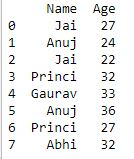Now we apply groupby() without sort

Python3

 # using groupby function # without using sort   df.groupby(['Name']).sum()

Output :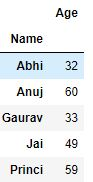Now we apply groupby() using sort in order to attain potential speedups

Python3

 # using groupby function # with sort   df.groupby(['Name'], sort = False).sum()

Output :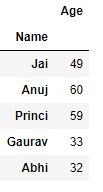Grouping data with object attributes :
Groups attribute is like dictionary whose keys are the computed unique groups and corresponding values being the axis labels belonging to each group.

Python3

 # importing pandas module import pandas as pd    # Define a dictionary containing employee data data1 = {'Name':['Jai', 'Anuj', 'Jai', 'Princi',                  'Gaurav', 'Anuj', 'Princi', 'Abhi'],         'Age':[27, 24, 22, 32,                33, 36, 27, 32],         'Address':['Nagpur', 'Kanpur', 'Allahabad', 'Kannuaj',                    'Jaunpur', 'Kanpur', 'Allahabad', 'Aligarh'],         'Qualification':['Msc', 'MA', 'MCA', 'Phd',                          'B.Tech', 'B.com', 'Msc', 'MA']}         # Convert the dictionary into DataFrame  df = pd.DataFrame(data1)    print(df)Now we group data like we do in a dictionary using keys.

Python3

 # using keys for grouping # data   df.groupby('Name').groups

Output :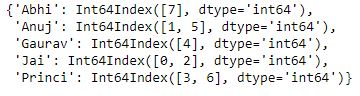Iterating through groups

In order to iterate an element of groups, we can iterate through the object similar to itertools.obj.

Python3

 # importing pandas module import pandas as pd    # Define a dictionary containing employee data data1 = {'Name':['Jai', 'Anuj', 'Jai', 'Princi',                  'Gaurav', 'Anuj', 'Princi', 'Abhi'],         'Age':[27, 24, 22, 32,                33, 36, 27, 32],         'Address':['Nagpur', 'Kanpur', 'Allahabad', 'Kannuaj',                    'Jaunpur', 'Kanpur', 'Allahabad', 'Aligarh'],         'Qualification':['Msc', 'MA', 'MCA', 'Phd',                          'B.Tech', 'B.com', 'Msc', 'MA']}         # Convert the dictionary into DataFrame  df = pd.DataFrame(data1)    print(df)Now we iterate an element of group in a similar way we do in itertools.obj.

Python3

 # iterating an element # of group   grp = df.groupby('Name') for name, group in grp:     print(name)     print(group)     print()

Output :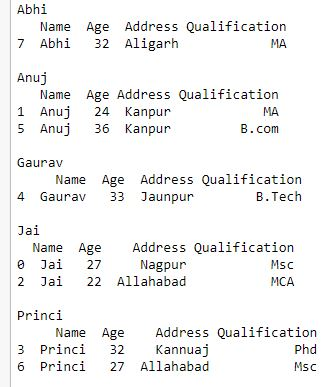Now we iterate an element of group containing multiple keys

Python3

 # iterating an element # of group containing # multiple keys   grp = df.groupby(['Name', 'Qualification']) for name, group in grp:     print(name)     print(group)     print()

Output :
As shown in output that group name will be tuple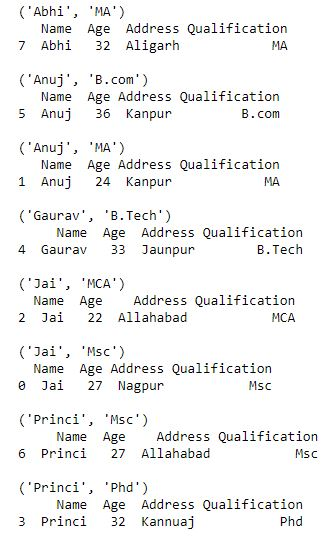Selecting a groups

In order to select a group, we can select group using GroupBy.get_group(). We can select a group by applying a function GroupBy.get_group this function select a single group.

Python3

 # importing pandas module import pandas as pd    # Define a dictionary containing employee data data1 = {'Name':['Jai', 'Anuj', 'Jai', 'Princi',                  'Gaurav', 'Anuj', 'Princi', 'Abhi'],         'Age':[27, 24, 22, 32,                33, 36, 27, 32],         'Address':['Nagpur', 'Kanpur', 'Allahabad', 'Kannuaj',                    'Jaunpur', 'Kanpur', 'Allahabad', 'Aligarh'],         'Qualification':['Msc', 'MA', 'MCA', 'Phd',                          'B.Tech', 'B.com', 'Msc', 'MA']}         # Convert the dictionary into DataFrame  df = pd.DataFrame(data1)    print(df)Now we select a single group using Groupby.get_group.

Python3

 # selecting a single group   grp = df.groupby('Name') grp.get_group('Jai')

Output :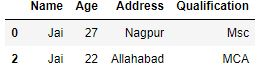Now we select an object grouped on multiple columns

Python3

 # selecting object grouped # on multiple columns   grp = df.groupby(['Name', 'Qualification']) grp.get_group(('Jai', 'Msc'))

Output :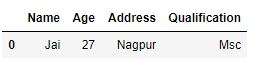Applying function to group

After splitting a data into a group, we apply a function to each group in order to do that we perform some operation they are:

• Aggregation : It is a process in which we compute a summary statistic (or statistics) about each group. For Example, Compute group sums ormeans
• Transformation : It is a process in which we perform some group-specific computations and return a like-indexed. For Example, Filling NAs within groups with a value derived from each group
• Filtration : It is a process in which we discard some groups, according to a group-wise computation that evaluates True or False. For Example, Filtering out data based on the group sum or mean

Aggregation :
Aggregation is a process in which we compute a summary statistic about each group. Aggregated function returns a single aggregated value for each group. After splitting a data into groups using groupby function, several aggregation operations can be performed on the grouped data.
Code #1: Using aggregation via the aggregate method

Python3

 # importing pandas module import pandas as pd   # importing numpy as np import numpy as np    # Define a dictionary containing employee data data1 = {'Name':['Jai', 'Anuj', 'Jai', 'Princi',                  'Gaurav', 'Anuj', 'Princi', 'Abhi'],         'Age':[27, 24, 22, 32,                33, 36, 27, 32],         'Address':['Nagpur', 'Kanpur', 'Allahabad', 'Kannuaj',                    'Jaunpur', 'Kanpur', 'Allahabad', 'Aligarh'],         'Qualification':['Msc', 'MA', 'MCA', 'Phd',                          'B.Tech', 'B.com', 'Msc', 'MA']}         # Convert the dictionary into DataFrame  df = pd.DataFrame(data1)    print(df)Now we perform aggregation using aggregate method

Python3

 # performing aggregation using # aggregate method   grp1 = df.groupby('Name')   grp1.aggregate(np.sum)

Output :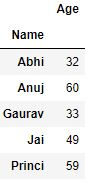Now we perform aggregation on agroup containing multiple keys

Python3

 # performing aggregation on # group containing multiple # keys grp1 = df.groupby(['Name', 'Qualification'])   grp1.aggregate(np.sum)

Output :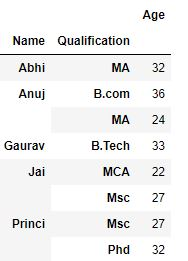Applying multiple functions at once :
We can apply a multiple functions at once by passing a list or dictionary of functions to do aggregation with, outputting a DataFrame.

Python3

 # importing pandas module import pandas as pd   # importing numpy as np import numpy as np    # Define a dictionary containing employee data data1 = {'Name':['Jai', 'Anuj', 'Jai', 'Princi',                  'Gaurav', 'Anuj', 'Princi', 'Abhi'],         'Age':[27, 24, 22, 32,                33, 36, 27, 32],         'Address':['Nagpur', 'Kanpur', 'Allahabad', 'Kannuaj',                    'Jaunpur', 'Kanpur', 'Allahabad', 'Aligarh'],         'Qualification':['Msc', 'MA', 'MCA', 'Phd',                          'B.Tech', 'B.com', 'Msc', 'MA']}         # Convert the dictionary into DataFrame  df = pd.DataFrame(data1)    print(df)Now we apply a multiple functions by passing a list of functions.

Python3

 # applying a function by passing # a list of functions   grp = df.groupby('Name')   grp['Age'].agg([np.sum, np.mean, np.std])

Output :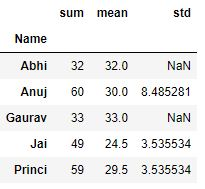Applying different functions to DataFrame columns :
In order to apply a different aggregation to the columns of a DataFrame, we can pass a dictionary to aggregate .

Python3

 # importing pandas module import pandas as pd   # importing numpy as np import numpy as np    # Define a dictionary containing employee data data1 = {'Name':['Jai', 'Anuj', 'Jai', 'Princi',                  'Gaurav', 'Anuj', 'Princi', 'Abhi'],         'Age':[27, 24, 22, 32,                33, 36, 27, 32],         'Address':['Nagpur', 'Kanpur', 'Allahabad', 'Kannuaj',                    'Jaunpur', 'Kanpur', 'Allahabad', 'Aligarh'],         'Qualification':['Msc', 'MA', 'MCA', 'Phd',                          'B.Tech', 'B.com', 'Msc', 'MA'],         'Score': [23, 34, 35, 45, 47, 50, 52, 53]}         # Convert the dictionary into DataFrame  df = pd.DataFrame(data1)    print(df)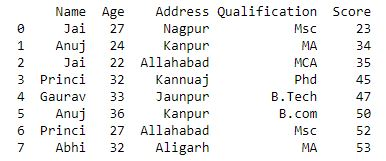Now we apply a different aggregation to the columns of a dataframe.

Python3

 # using different aggregation # function by passing dictionary # to aggregate grp = df.groupby('Name')   grp.agg({'Age' : 'sum', 'Score' : 'std'})

Output :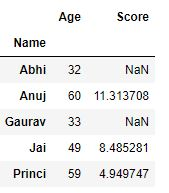Transformation :
Transformation is a process in which we perform some group-specific computations and return a like-indexed. Transform method returns an object that is indexed the same (same size) as the one being grouped. The transform function must:

• Return a result that is either the same size as the group chunk
• Operate column-by-column on the group chunk
• Not perform in-place operations on the group chunk.

Python3

 # importing pandas module import pandas as pd   # importing numpy as np import numpy as np    # Define a dictionary containing employee data data1 = {'Name':['Jai', 'Anuj', 'Jai', 'Princi',                  'Gaurav', 'Anuj', 'Princi', 'Abhi'],         'Age':[27, 24, 22, 32,                33, 36, 27, 32],         'Address':['Nagpur', 'Kanpur', 'Allahabad', 'Kannuaj',                    'Jaunpur', 'Kanpur', 'Allahabad', 'Aligarh'],         'Qualification':['Msc', 'MA', 'MCA', 'Phd',                          'B.Tech', 'B.com', 'Msc', 'MA'],         'Score': [23, 34, 35, 45, 47, 50, 52, 53]}         # Convert the dictionary into DataFrame  df = pd.DataFrame(data1)    print(df)Now we perform some group-specific computations and return a like-indexed.

Python3

 # using transform function grp = df.groupby('Name') sc = lambda x: (x - x.mean()) / x.std()*10 grp.transform(sc)

Output :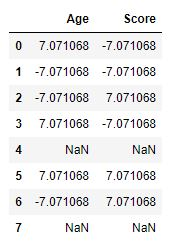Filtration :
Filtration is a process in which we discard some groups, according to a group-wise computation that evaluates True or False. In order to filter a group, we use filter method and apply some condition by which we filter group.

Python3

 # importing pandas module import pandas as pd   # importing numpy as np import numpy as np    # Define a dictionary containing employee data data1 = {'Name':['Jai', 'Anuj', 'Jai', 'Princi',                  'Gaurav', 'Anuj', 'Princi', 'Abhi'],         'Age':[27, 24, 22, 32,                33, 36, 27, 32],         'Address':['Nagpur', 'Kanpur', 'Allahabad', 'Kannuaj',                    'Jaunpur', 'Kanpur', 'Allahabad', 'Aligarh'],         'Qualification':['Msc', 'MA', 'MCA', 'Phd',                          'B.Tech', 'B.com', 'Msc', 'MA'],         'Score': [23, 34, 35, 45, 47, 50, 52, 53]}         # Convert the dictionary into DataFrame  df = pd.DataFrame(data1)    print(df)Now we filter data that to return the Name which have lived two or more times .

Python3

 # filtering data using # filter data grp = df.groupby('Name') grp.filter(lambda x: len(x) >= 2)

Output :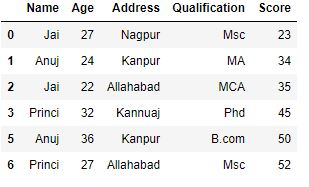My Personal Notes arrow_drop_up
Recommended Articles
Page :